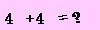﻿ MM 850/1310nm Coupler
Hot products
•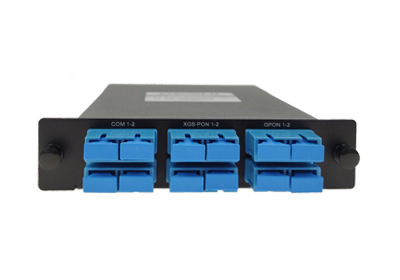•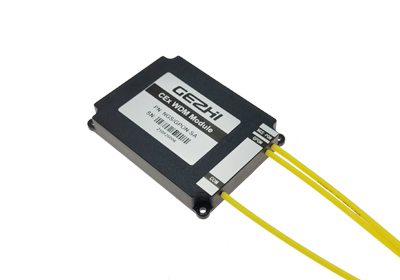•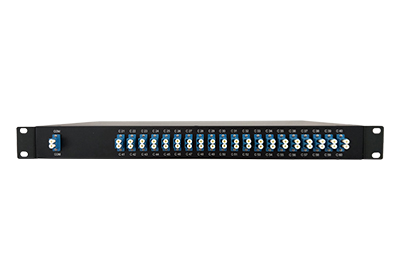•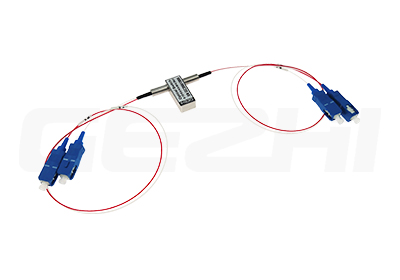Related Products
No Info in this class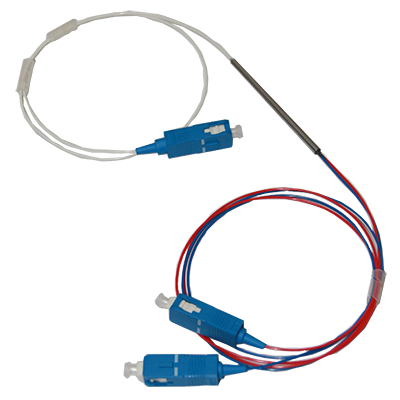# MM 850/1310nm Coupler

MM 850/1310nm Coupler
•  MM 850/1310nm Coupler

Decriptions

Gezhi Photonics' Singlemode wideband coupler can make the optical singal re-coupled in the special couple area during the transmission, then reassigned the optical power. Optional Coupling ratio.The Singlemode Optic-fiber Tree and Star Couplers can couple the optical signals in the coupling area, and then re-distribute the light power. The key parameters include operating wavelength, bandwidth, and excess loss, coupling ratio, PDL and so on.

Features

Low Insertion loss

High Return Loss

Option Coupling Ratio

Wide Operating Temperature

Excellent Environmental & Mechanial Stability

Applications

♦         FTTX Systems

PON Networks

Local Area Networks (LAN)

Test Equipments

Compliance

Telcordia GR-1209-CORE-2001

Telcordia GR-1221-CORE-1999

RoHS

Technical Paremeter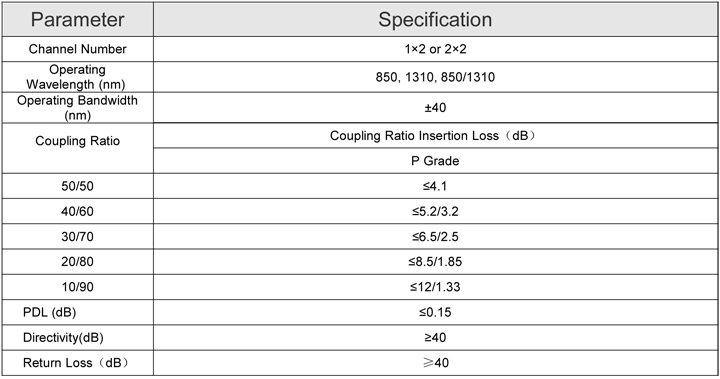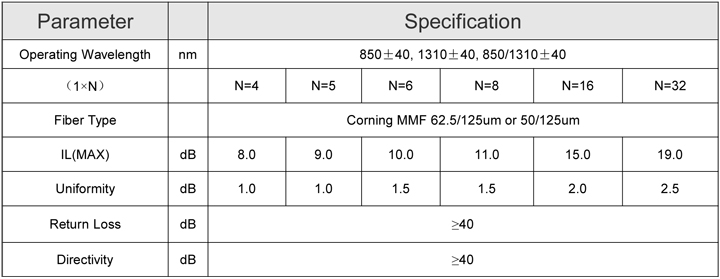Ordering information

 GC XXX X XX XX XX XX Port Configrition Module type Cable type Fiber length Input connector Outputconnector G=GezhiC=Coupler 102=1x2102=1x3104=1x4108=1x8116=1x16132=1x32204=2x4208=2x8216=2x16232=2x32 0=None1=￠3x54mm2=90x20x10mm3=100x80x10mm4=120x80x18mm5=140x115x186=LGX Box7=19″1U RackX=Customized 00=none09=900umLoose tube20=2.0mm30=3.0mm 05=0.5m10=1.0m15=1.5m30=3.0m40=4.0m50=5.0m...... 0=none1=LC/UPC2=LC/APC3=SC/UPC4=SC/APC5=FC/UPC6=FC/APC7=LC/APCX=Customized 0=none1=LC/UPC2=LC/APC3=SC/UPC4=SC/APC5=FC/UPC6=FC/APC7=LC/APCX=Customized
• ## verification code